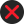Procedure to be followed in calculating interest. [12. In calculating the interest payable by the assessee or the interest payable by the Central Government to the assessee under any provision of the Act,— (a)     where interest is to be calculated on annual basis, the period for which such interest is to be calculated shall be rounded off to a whole month or months and for this purpose any fraction of a month shall be ignored; and the period so rounded off shall be deemed to be the period in respect of which the interest is to be calculated; (b)     where the interest is to be calculated for every month or part of a month comprised in a period, any fraction of a month shall be deemed to be a full month and the interest shall be so calculated; (c)        the amount of tax, penalty or other sum in respect of which such interest is to be calculated shall be rounded off to the nearest multiple of one hundred rupees and for this purpose any fraction of one hundred rupees shall be ignored; and the amount so rounded off shall be deemed to be the amount in respect of which the interest is to be calculated.]